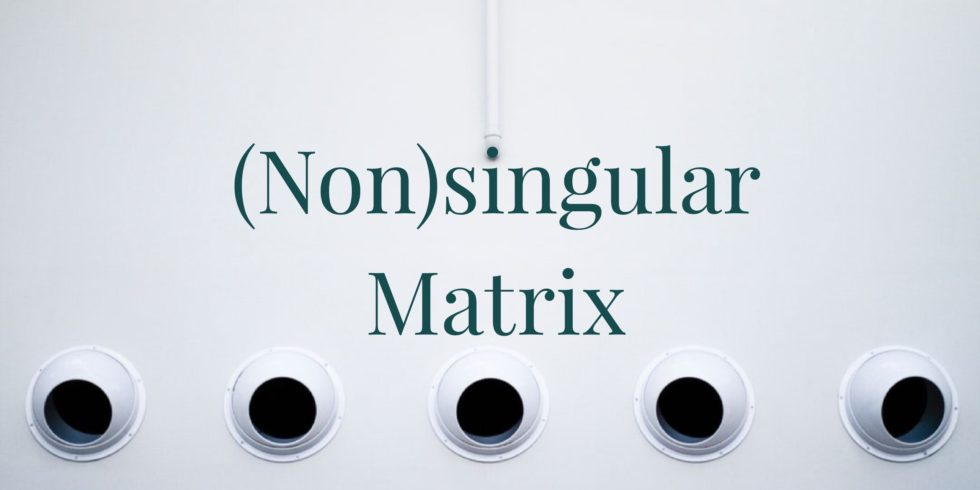# If $M, P$ are Nonsingular, then Exists a Matrix $N$ such that $MN=P$## Problem 657

Suppose that $M, P$ are two $n \times n$ non-singular matrix. Prove that there is a matrix $N$ such that $MN = P$.Add to solve later

## Proof.

As non-singularity and invertibility are equivalent, we know that $M$ has the inverse matrix $M^{-1}$.

Let us think backwards. Suppose that we have $MN=P$ for some matrix $N$, which we want to find.
Then multiply $M^{-1}$ on the left of the equation $MN = P$ yields $N = M^{-1} P$.

This is the matrix we are looking for, as $M N = M ( M^{-1} P) = P$.Add to solve later

### More from my site

#### You may also like...

This site uses Akismet to reduce spam. Learn how your comment data is processed.

###### More in Linear Algebra##### A Condition that a Vector is a Linear Combination of Columns Vectors of a Matrix

Suppose that an $n \times m$ matrix $M$ is composed of the column vectors $\mathbf{b}_1 , \cdots , \mathbf{b}_m$. Prove...

Close